• 雅可比法用于对称矩阵，QR用于非对称...QR分解法求特征向量及其特征值c++程序源于feitian_feiwu的资源，本资源增加了复特征值对应的特征向量计算。 vs2013下调试通过，复特征向量不唯一，程序里提供了在线验证网址，
• 关于矩阵运算的各种数值算法，包括实（）矩阵求逆，对称正定矩阵与托伯利兹矩阵的求逆，线性方程组的常用解法，矩阵的各种分解方法，特征向量特征值的求解等等。
• 1、设T是数域K上的线性空间的线性变换，且对K中某一数,存在非零向量,使得    成立，则称为T的特征值，x为T的...4、复数域上n*n矩阵A的n个特征值的几何意义是平面上的n个点 5、特征值的估计   更新中。。。...
1、设T是数域K上的线性空间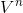的线性变换，且对K中某一数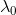,存在非零向量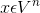,使得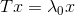成立，则称为T的特征值，x为T的属于的特征向量

2、特征矩阵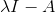3、特征多项式为A的特征矩阵的行列式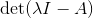4、复数域上n*n矩阵A的n个特征值的几何意义是复平面上的n个点

5、特征值的估计

更新中。。。
展开全文矩阵论 线性代数
• Hermite矩阵求特征值特征向量的问题转化为求解实对称阵的特征值特征向量
• 0. 我们可以将特征值特征向量类比于信号与系统课程中的特征函数。在那里，系统对特征函数的作用相当于乘以一个（）常数。  于是，我们可以将矩阵A想象为一个“系统”，输入到该系统的“信号”x可分解为特征...
[作者：byeyear，首发于cnblogs.com，转载请注明。联系：east3@163.com]
0. 我们可以将特征值与特征向量类比于信号与系统课程中的特征函数。在那里，系统对特征函数的作用相当于乘以一个（复）常数。
于是，我们可以将矩阵A想象为一个“系统”，输入到该系统的“信号”x可分解为特征向量的加权和，
这样，矩阵A这个“系统”对任意向量x的作用就可分解为A系统对特征向量作用的加权和。
1. 一个例子：随机矩阵与其稳态向量q满足Aq=q，此时 特征值λ=1，特征向量即稳态向量q。
2. 由Ax=λx，可以推出(A-λI)x=0。由于特征向量不能为0，该方程必须有非平凡解，因此A-λI不可逆；
所以det(A-λI)=0。据此可解出所有λ，再跟据λ可解出特征向量。det(A-λI)=0称为特征方程。
3. 根据齐次线性方程组的特点，一个特征值对应的特征向量有无限多个，且对应于λ的所有特征向量加上零向量可以构成向量空间，称为矩阵A对应于特征值λ的特征空间。
4. n阶矩阵的特征方程是λ的n阶方程，如果将特征向量限制在R中，那么特征方程未必有解，即不是所有的矩阵都有R域中的特征值；但每一个矩阵一定存在n个复数域中的特征值（k重根按k个特征值计）。
5. 设λ1,...λr是n阶矩阵的r个相异特征值，v1,...vr是对应的r个特征向量，那么向量集{v1,...vr}线性无关。
6. 若存在可逆矩阵P使得两个n阶矩阵A，B满足A=PBP-1，则称A，B相似。
7. 若n阶矩阵A，B相似，那么这两者有相同的特征多项式，从而有相同的特征值（包括相同的代数重数）。但特征向量一般不同！！！
8. 特征向量的应用举例
假设我们现在要分析xk+1=Axk，x0已知。如果我们能将x0分解为A的特征向量的线性组合，比如x0=c1v1+c2v2，v1，v2为A的特征向量，
那么上述递归方程就能有一个简单的解法解出xk：
x1=Ax0
=A(c1v1+c2v2)
=c1Av1+c2Av2
=c1λv1+c2λv2
x2=Ax1
=A(c1λv1+c2λv2)
=c1λAv1+c2λAv2
=c1λ2v1+c2λ2v2
...
xk=c1λkv1+c2λkv2
9. n阶矩阵可对角化的条件是A有n个线性无关的特征向量。
若A=PDP-1，D为对角阵，那么P的列向量是A的n个线性无关的特征向量，D的主对角线元素是A的对应于P中特征向量的特征值。
换言之，A可对角化的充分必要条件是有足够多的特征向量形成Rn的基。这样的基称为特征向量基。
10. 某特征值对应的特征空间的维数小于或等于该特征值的代数重数。
11. 矩阵A可对角化的充分必要条件是所有不同特征空间的维数和为n，即每个特征值对应特征空间的维数等于该特征值的代数重数。
12. 若A可对角化，那么所有特征空间的基的向量的集合是Rn的特征向量基。
13. 若V是n维向量空间，W是m维向量空间，T是V到W的线性变换，B和C分别是V和W的基，那么T相对于基B和C的矩阵为：
M=[ [T(b1)]c  ...  [T(bn)]c ]
用M来表示V到W的变换：
[T(x)]c = M[x]B
直观的解释：V中的任意一个向量都能表示为基B中各向量的线性组合，因此，只要知道了B中各向量经变换T后在W中的“样子”，就能知道V中任意向量经变换T后在W中的“样子”。
若W=V，C=B，上式即简化为：
[T(x)]B = [T]B[x]B
此时M=[T]B，称为T相对于B的矩阵，或简称为T的B-矩阵。
14. 设A=PDP-1，D为n阶对角矩阵，若Rn的基由P的列向量组成，那么D是变换x|->Ax的B-矩阵。
写成表达式就是，若x|->Ax，那么[x]B|->D[x]B，[x]B是x在P的列向量所组成的基下的坐标。
上面两个变换式x|->Ax和[x]B|->D[x]B描述的是相对于不同基的同一个线性变换。
这也解释了什么叫做“相似”：两个相似矩阵可用来描述（相对于不同基的）同一个线性变换。
实际上，上述表述中，D不一定要是对角矩阵。
设y=Ax，且A可表示成PDP-1，那么：
y=Ax
=> y=PDP-1x
=> P-1y=P-1PDP-1x
=> P-1y=DP-1x
=> (P-1y)=D(P-1x)
P-1y和P-1x可分别看作y和x在Rn的基P下的坐标。
对于差分方程xk+1=Axk，上式就成为：
P-1xk+1=D(P-1xk)
用w表示x在P下的坐标，就是：
wK+1=Dwk
矩阵A对角化后的最大优点是解耦了向量x的各分量。如果A可对角化，那么在A的特征向量基下，运算Ax将简化为各方向上的缩放（Du）。
15. 
展开全文• 1. 共轭复特征值 设AAA是n×nn\times nn×n的实矩阵， Ax‾=Aˉxˉ=Axˉ \overline{Ax}=\bar{A}\bar{x}=A\bar{x} Ax=Aˉxˉ=Axˉ 假设λ\lambdaλ是AAA的特征值，xxx为λ\lambdaλ对应的特征向量，则λˉ\bar{\...
1. 共轭复特征值
设$A$是$n\times n$的实矩阵，
$\overline{Ax}=\bar{A}\bar{x}=A\bar{x}$
假设$\lambda$是$A$的特征值，$x$为$\lambda$对应的特征向量，则$\bar{\lambda}$同样是$A$的特征值，而$\bar{x}$是对应的特征向量，
$A\bar{x}=\overline{Ax}=\overline{\lambda x}=\bar{\lambda}\bar{x}$
所以，当$A$是$n\times n$的实矩阵，它的复特征值以共轭复数对出现。
2. rotation-scaling matrix
假如$a$,$b$为实数，且不同时为0，则将下面的矩阵称为rotation-scaling matrix，
$A=\begin{bmatrix} a & -b \\ b & a \end{bmatrix} \tag{1}$
则有，

A可以写成下面的旋转+缩放形式，
\begin{aligned} A&=\begin{bmatrix} a & -b \\ b & a \end{bmatrix}\\ &=\begin{bmatrix} r &0 \\ 0 &r \\ \end{bmatrix} \begin{bmatrix} \frac{a}{r} & \frac{-b}{r}\\ \frac{b}{r}& \frac{a}{r} \end{bmatrix} \\ &=\begin{bmatrix} r &0 \\ 0 &r \\ \end{bmatrix} \begin{bmatrix} \cos(\theta)& -\sin(\theta)\\ \sin(\theta)& \cos(\theta) \end{bmatrix} \\ \end{aligned} \tag{2}
其中，$r=\sqrt{\det(A)}=\sqrt{a^2+b^2}$，则$A$先旋转$\theta$，再倍乘$r$。

$A$的特征值为$\lambda=a\pm ib$。3. 矩阵的复特征值
首先我们假定下面的记号，
\begin{aligned} Re(a + bi) = a \\ Im(a + bi) = b \\ \operatorname{Re}\left(\begin{array}{l} x+y i \\ z+w i \end{array}\right)=\left(\begin{array}{l} x \\ z \end{array}\right) \\ \operatorname{Im}\left(\begin{array}{l} x+y i \\ z+w i \end{array}\right)=\left(\begin{array}{l} y \\ w \end{array}\right) \end{aligned} \tag{3}
这里首先讨论的矩阵是$2\times2$的实矩阵，且矩阵有复特征值$\lambda$，而与特征值相对应的特征向量为$v$，这时候有个很漂亮的结论$A=CBC^{-1}$，其中
$C=\left(\begin{array}{cc} | & | \\ \operatorname{Re}(v) & \operatorname{Im}(v) \\ | & | \end{array}\right) \quad \text { and } \quad B=\left(\begin{array}{cc} \operatorname{Re}(\lambda) & \operatorname{Im}(\lambda) \\ -\operatorname{Im}(\lambda) & \operatorname{Re}(\lambda) \end{array}\right)\tag{4}$
其中$B$矩阵为rotation-scaling matrix。
为了证明矩阵$A$的分解公式成立，我们首先证明$C$是可逆的，即$\operatorname{Re}(v)$和$\operatorname{Im}(v)$是线性无关的。用反证法，假设$\operatorname{Re}(v)$和$\operatorname{Im}(v)$是线性相关的，则存在$x,y$，使得，$x\operatorname{Re}(v)+y\operatorname{Im}(v)=0$，则
\begin{aligned} (y+i x) v &=(y+i x)(\operatorname{Re}(v)+i \operatorname{Im}(v)) \\ &=y \operatorname{Re}(v)-x \operatorname{Im}(v)+(x \operatorname{Re}(v)+y \operatorname{Im}(v)) i \\ &=y \operatorname{Re}(v)-x \operatorname{Im}(v) \end{aligned}\tag{5}
$(y+i x) v$依然是属于特征值$\lambda$的特征向量，而从式(5)可以得到$(y+i x) v$是个实向量，而对于一个实矩阵的实特征向量对应的特征值一定是实的，但是和$\lambda$是复特征根矛盾，因此可证$\operatorname{Re}(v)$和$\operatorname{Im}(v)$是线性无关的。
此外，我们假设复特征值$\lambda=a+bi$，同时对应的特征向量为$v=\begin{pmatrix} x+yi \\ z+wi \end{pmatrix}$，则有，
\begin{aligned} A v=\lambda v &=(a+b i)\left(\begin{array}{c} x+y i \\ z+w i \end{array}\right) \\ &=\left(\begin{array}{c} (a x-b y)+(a y+b x) i \\ (a z-b w)+(a w+b z) i \end{array}\right) \\ &=\left(\begin{array}{c} a x-b y \\ a z-b w \end{array}\right)+i\left(\begin{array}{c} a y+b x \\ a w+b z \end{array}\right) \end{aligned}\tag{6}
同时，
$A\left(\left(\begin{array}{l} x \\ z \end{array}\right)+i\left(\begin{array}{l} y \\ w \end{array}\right)\right)=A\left(\begin{array}{l} x \\ z \end{array}\right)+i A\left(\begin{array}{l} y \\ w \end{array}\right)=A \operatorname{Re}(v)+i A \operatorname{Im}(v)\tag{7}$
比较式(6)和(7)，可以得到，
$\operatorname{ARe}(v)=\left(\begin{array}{l} a x-b y \\ a z-b w \end{array}\right) \quad \operatorname{AIm}(v)=\left(\begin{array}{l} a y+b x \\ a w+b z \end{array}\right)\tag{8}$
接下来我们计算$C B C^{-1} \operatorname{Re}(v)$，和$C B C^{-1} \operatorname{Im}(v)$，由(4)式可以马上得到$Ce_1=\operatorname{Re}(v)$,$Ce_2=\operatorname{Im}(v)$(自然基取对应的列)，则有
\begin{aligned} C B C^{-1} \operatorname{Re}(v) &=C B e_{1}=C\left(\begin{array}{c} a \\ -b \end{array}\right)=a \operatorname{Re}(v)-b \operatorname{Im}(v) \\ &=a\left(\begin{array}{l} x \\ z \end{array}\right)-b\left(\begin{array}{l} y \\ w \end{array}\right)=\left(\begin{array}{l} a x-b y \\ a z-b w \end{array}\right)=A \operatorname{Re}(v) \\ C B C^{-1} \operatorname{Im}(v) &=C B e_{2}=C\left(\begin{array}{l} b \\ a \end{array}\right)=b \operatorname{Re}(v)+a \operatorname{Im}(v) \\ &=b\left(\begin{array}{l} x \\ z \end{array}\right)+a\left(\begin{array}{l} y \\ w \end{array}\right)=\left(\begin{array}{c} a y+b x \\ a w+b z \end{array}\right)=A \operatorname{Im}(v) \end{aligned}\tag{9}
因为$\operatorname{Re}(v)$和$\operatorname{Im}(v)$的线性无关的，可以组成$\mathbb{R}^2$的基，对于任意的向量$w$，$w=c\operatorname{Re}(v)+d\operatorname{Im}(v)$，则有，
\begin{aligned} A w &=A(\operatorname{cRe}(v)+d \operatorname{Im}(v)) \\ &=\operatorname{cARe}(v)+d A \operatorname{Im}(v) \\ &=c C B C^{-1} \operatorname{Re}(v)+d C B C^{-1} \operatorname{Im}(v) \\ &=C B C^{-1}(c \operatorname{Re}(v)+d \operatorname{Im}(v)) \\ &=C B C^{-1} w \end{aligned}\tag{10}
因此$A=C B C^{-1}$。
对于$A$的带有rotation-scaling matrix的分解，我们可以这么理解，$A$中含有旋转和比例变换，矩阵$C$提供了变量代换，如$x=Cu$。$A$的作用相当于先将$x$代换为$u$，然后在$C$所形成的基下利用$B$矩阵进行旋转和缩放，旋转产生一个椭圆，然后将$u$再变量代换回$x$。注意，旋转是在$C$所形成的基下，即顺着$\operatorname{Re}(v)$和$\operatorname{Im}(v)$所形成的基旋转。
对于$n\times n$矩阵，都有类似上述$2\times 2$矩阵的分解形式，下面以$3\times 3$为列，如果矩阵$A$有一个实的特征值$\lambda_{2}$，一个复特征值$\lambda_{1}$，则$\overline{\lambda_{1}}$为另外一个复特征值，$\lambda_{2}$对应的实特征向量为$v_2$，$\lambda_{1}$对应的复特征向量为$v_1$，将$A$分解为$A=C B C^{-1}$,
$C=\left(\begin{array}{ccc} | & | & | \\ \operatorname{Re}\left(v_{1}\right) & \operatorname{Im}\left(v_{1}\right) & v_{2} \\ | & | & | \end{array}\right) \quad B=\left(\begin{array}{ccc} \operatorname{Re}\left(\lambda_{1}\right) & \operatorname{Im}\left(\lambda_{1}\right) & 0 \\ -\operatorname{Im}\left(\lambda_{1}\right) & \operatorname{Re}\left(\lambda_{1}\right) & 0 \\ \hline 0 & 0 & \lambda_{2} \end{array}\right)\tag{11}$
对于上述矩阵$A$，在$\mathbb{R}^{3}$中存在某个平面$A$对平面的作用是旋转和缩放，该平面在$A$的作用下是不变的。
举一个例子，例如，
$A=\begin{bmatrix} 0.8 & -0.6 & 0 \\ 0.6 & 0.8 & 0 \\ 0 & 0 & 1.07 \end{bmatrix}$
上述矩阵$A$与式(11)中的矩阵形式相同，如下图所示，对于$x_1x_2$平面(第三坐标为0)的任一向量$w_0$被$A$旋转到该平面的另外一个位置上，不在该平面的任一向量$x_0$的第三坐标乘1.07。下图显示了$w_0=(2,0,0)$和$x_0=(2,0,1)$被$A$作用的迭代结果，$w_0$在$x_1x_2$平面旋转，而$x_0$在乘1.07后在旋转的同时也在盘旋上升。展开全文自动驾驶
• 该程序求解了hermit矩阵的特征值特征向量
• 机器学习教程「美团」算法工程师带你入门机器学习 已经开始更新了，欢迎大家订阅~ 任何关于算法、编程、AI行业知识或博客内容问题，可以随时扫码关注公众号「图灵猫」，加入”学习小组“，沙雕博...今天...
任何关于算法、编程、AI行业知识或博客内容的问题，可以随时扫码关注公众号「图灵的猫」，加入”学习小组“，沙雕博主在线答疑~此外，公众号内还有更多AI、算法、编程和大数据知识分享，以及免费的SSR节点和学习资料。其他平台（知乎/B站）也是同名「图灵的猫」，不要迷路哦~今天复习了一下线性代数，突然发现自己把最简单的东西都给忘了。。赶紧整理一下，其实这两个概念用三句话就能说清：

特征向量：就是在某个线性变换下方向不变（也可以说具有保角性），其大小不变或乘以某个缩放因子的非零向量。线性变换的主特征向量是最大特征值对应的特征向量。

特征值：就是上面说的那个缩放因子了，一般都是从特征方程算出来的（叫特征根），是变换的本质

特征空间：就是由所有有着相同特征值的特征向量组成的空间，还包括零向量，但要注意零向量本身不是特征向量。

谱：其实就是特征值了，谱分解也和上面这三个东西有关。有空我们再细细讨论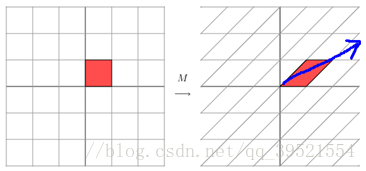展开全文线性代数 统计学习 矩阵分析 数学
• 目录Real and Imaginary Parts of Vectors 向量的实部和虚部Eigenvalues and Eigenvectors of a Real Matrix That Acts on Cn\mathbb C^nCn This section shows that if the characteristic equation of a real ...
• 利用四元数矩阵的表示及友向量的概念结合复数域上的 Hermitian阵的性质证明了四元数 自共轭矩阵的特征值的变分特征,并利用变分特征研究了四元数矩阵特征值的性质 。得到了四元数矩阵的 Wey1定理、单调性定理、柯西...
• 四元数矩阵右特征值的计算可转化为它的表示矩阵的特征值的计算问题，本文利用表示矩阵的特殊结构给出了一种减少计算其特征值计算量的方法。 四元数矩阵计算中有一些新的问题是矩阵计算中没有的内容。例如...
• #示例:S为特征向量构成矩阵,Λ为特征值构成对角矩阵 S^{-1}·A·S=Λ⇒A~Λ #性质: 1.A~B,B~C⇒A~C 2.A~B⇒A与B的特征值相同 Ax=λx⇒A·M·M^{-1}x=λx⇒M^{-1}·A·M·M^{-1}x=λM^{-1}x⇒B·M^{-1}x=λM^{-...线性代数
• 给出了一组可对原来方程进行自由度缩减向量基,然后与 Foss变换相结合,得到一组实用向量基,使之适用于求解复杂非比例阻尼线性系统任意低阶复振型和相应的复特征值,适用于任意扰力作用下动力反应分析。...
• 1. 特征子空间性质： 1）非空（至少包含零向量）且不等于零向量（即，包含零向量以外非零向量） 2）加法封闭 3）对数乘封闭 ...2. 要解决一些问题：判断、求解、应用特征值特征向量 ...
• 这里的λ就是你刚才解出来的特征根，整个意思是要你写成r(t)=c1e^λ1t+c2eλt的形式，那个c1,c2求法如上，等号右侧关于λ的矩阵是一个范德蒙矩阵的逆矩阵，乘以一个关于初始值的向量——而这两者都是已知的
• 提出一种英文文本检索算法，从文本中提取关键词项，根据转移概率计算出关键词项状态矩阵，并通过奇异分解，提取第一奇异向量作为复特征向量，利用向量间余弦相似度作为文本检索相似度度量。实验结果表明，...
• 在减压阀故障诊断实验中，对特定故障信号和正常信号同时采用相同耦合方式，根据二维小波可以有效提取信号中频率信息特点，利用二维小波提取双谱中这些耦合信息的特征值，并将这些特征值输入支持向量机进行...
• 研究射影空间中拟全实极小子流形谱几何,利用活动标架法并通过计算平均曲率向量的Laplacian,建立了仅与子流形内蕴几何量有关的特征值不等式,将相关结果推广到射影空间中一般子流形上,并获得了拟全实极小子...
• A可看作是角频率张量矩阵，由模态矩阵进行坐标变换解耦后可以得到所有模态参数，特征向量（模态系数）为复数不仅影响幅值，还影响相位角，而特征值为复数，其虚部为阻尼频率，而实部会使振动随时间衰减。...
• 利用小波变换提取发生故障后各支路零序电流暂态信号特征,将其作为改进RBF神经网络输入向量,再利用RBF神经网络强大自适应、自学习能力,对特征量进行训练,确保其快速收敛性以及选线准确性。仿真实验结果,...
• 全书共分15章和一个附录，主要包括函数微分学与积分学，幂级数理论及Laurent展开，残数理论及幅角原理，解析函数最大模原理及调和函数极值原理，解析函数唯一性定理及零点理论，整函数与半纯函数，Riemann...
• 把复数对角矩阵转化为实数块对角矩阵，如果特征方程[V,D]=eig(X)有成对的复特征值，cdf2cdf把矩阵V、D转化为实对角形式，对角线上2×2实数块将取代原有复数对。 【实例3.64】求矩阵x=[1,2,3;0,4,5;0,-5,4]...
• 全书共分15章和一个附录，主要包括函数微分学与积分学，幂级数理论及Laurent展开，残数理论及幅角原理，解析函数最大模原理及调和函数极值原理，解析函数唯一性定理及零点理论，整函数与半纯函数，Riemann...
• unique_values_viewer 一个QGIS插件，用于显示和复制矢量图层字段唯一。... “仅所选功能”选框可验证仅显示所选功能的值。 随着自动更新功能，该将交互地改变，当选择更改。 变更日志v0.2Python
• 7. 2 酉空间与酉矩阵7.2.1 酉空间7.2.2 酉矩阵正交矩阵的实标准形 7.2.1 酉空间 7.2.2 酉矩阵 对角化方法：计算特征向量（施密特正交化）并...先排列特征值为实数1，-1的特征向量，再排列复特征值特征向量 ...
• 复数矩阵及其应用矩阵 矩阵 对于实矩阵而言，要想使得特征值都是实数，且存在相互正交的特征向量，需要A=ATA=A^TA=AT 而对于矩阵，同样的要求需要AˉT=A\bar A^T=AAˉT=A ...线性代数
• 其内容包括插值与逼近，数值微分与数值积分，非线性方程与线性方程组数值解法，矩阵的特征值特征向量计算，常微分方程数值解法。每章附有习题并在书末给出了部分答案，每章还附有复习与思考题和计算实习题。全书...
• 比如说线性电路会将输入的正弦信号处理后，输出仍然是正弦信号，只是幅度和相位有一个变化（实际上从数学上看是因为指数函数是线性微分方程的特征函数，就好像矩阵的特征向量一样，而这个幅度对应特征值）。...
• 线性代数中，矩阵是避免不了话题，因为一个简单实矩阵都有可能有复数特征值矩阵 我们着重看一下矩阵和实矩阵在运算上区别。 距离 首先，一个复数向量的的距离求法发生了变化 x⊤x  → ...
• 如：矩阵加减乘除、转置、逆运算、 矩阵乘法、求行列式值、 求矩阵秩、一般实矩阵奇异值分解、求广义逆、约化对称矩阵为对称三对角阵、实对称三对角阵全部特征值特征向量的计算、求赫申伯格矩阵全部特征...